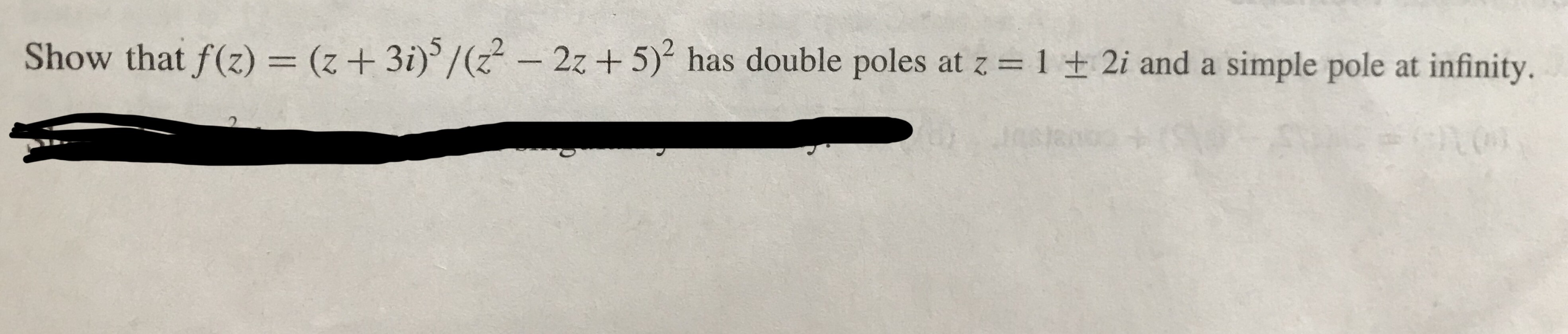# Show that f(z)(3i)/(22 -2z +5) has double poles at z 1 2i and a simple pole at infinity

Question
50 views

z is a complex variable z=x+iy

pic attached with questionhelp_outlineImage TranscriptioncloseShow that f(z)(3i)/(22 -2z +5) has double poles at z 1 2i and a simple pole at infinity fullscreen
check_circle

Step 1

To analyze and classify the singular points of the given function of the complex variable z

Step 2

Now, f(z) is a rational function (quotient of two polynomials) g(z)/h(z) . So the only singularities of f(z) in the finite part of the plane occur where h(z) =0 (poles counted with multiplicity). So, solve the equation h(z)=0

Step 3

As h(z) vanishes at these points with multiplicity two, ...

### Want to see the full answer?

See Solution

#### Want to see this answer and more?

Solutions are written by subject experts who are available 24/7. Questions are typically answered within 1 hour.*

See Solution
*Response times may vary by subject and question.
Tagged in